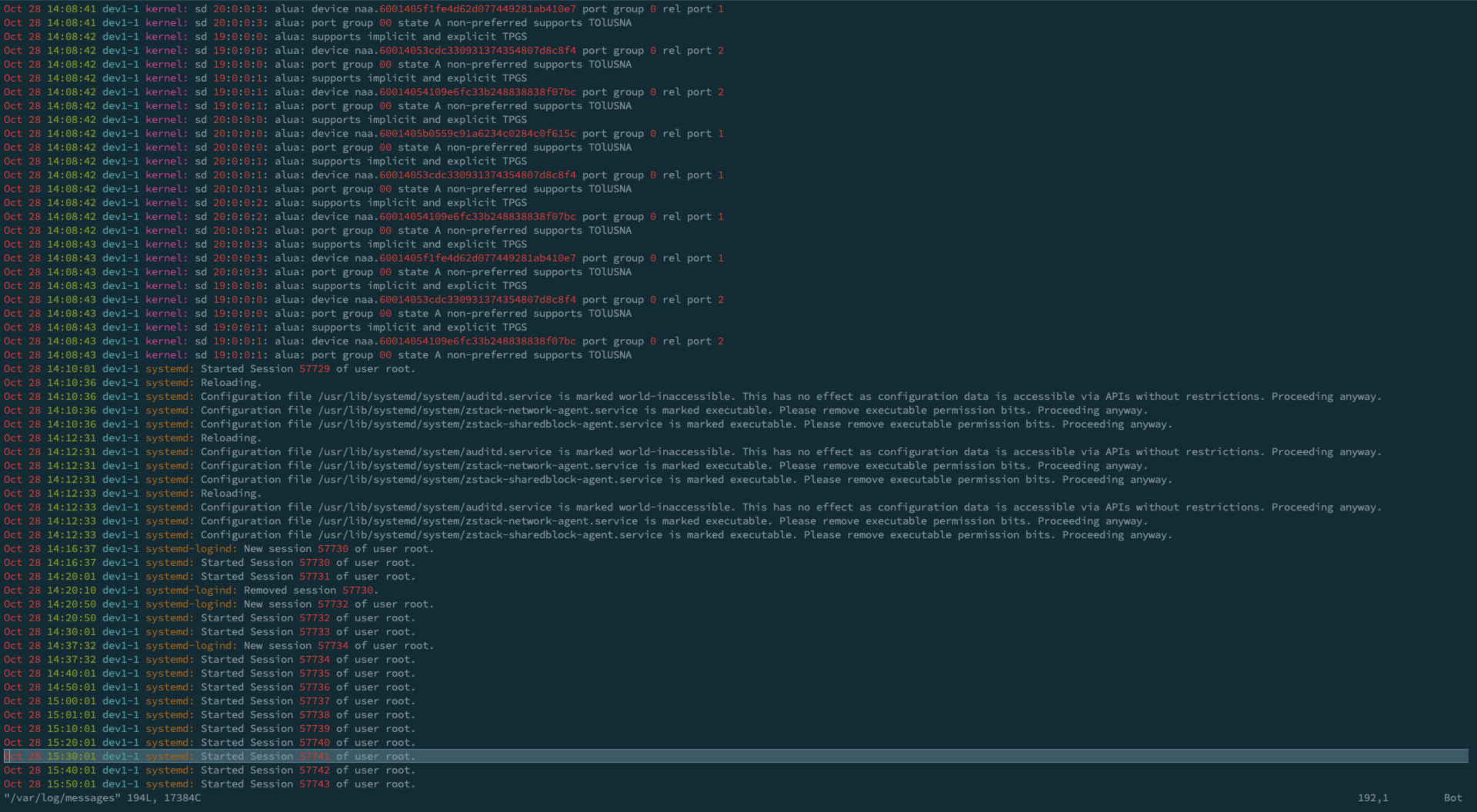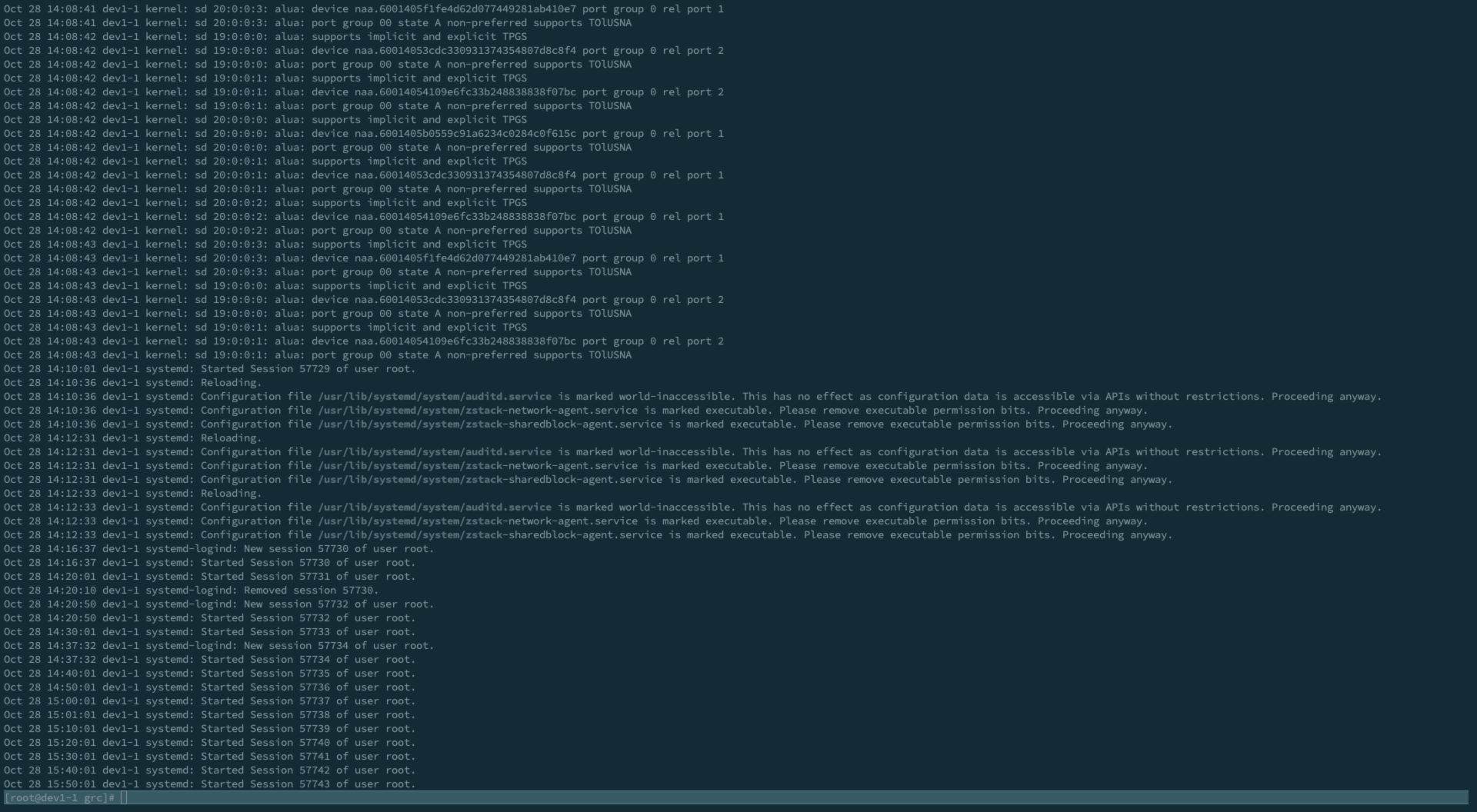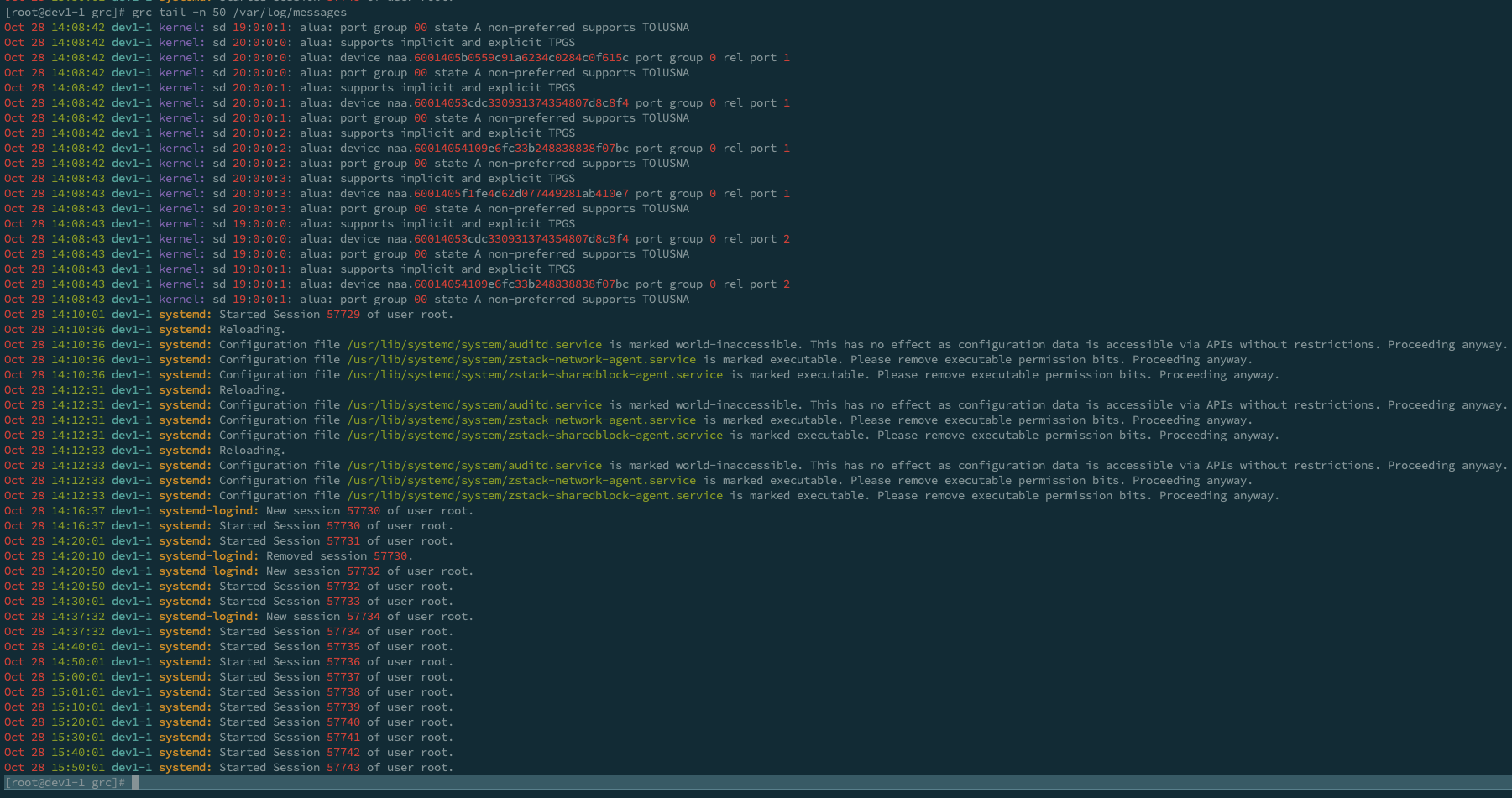# colored tail -f with /var/log/messages

Vim has beautiful color profile for messages:But I usually use tail -f to monitor my logs, while it can not use vim color profile, so I installed grc.
Clone and run `./install.sh`, try `grc tail -f /var/log/messages` and you will see:Oh, that’s not what I want.

Luckily grc can easyly customize profile, so this is my final effect:And this is my profile, play and enjoy 😀

``````[root@dev1-1 grc]# cat /usr/local/share/grc/conf.log
# this configuration file is suitable for displaying kernel log files

# example of text substitution
#regexp=\bda?emon
#replace=angel
#colours=red
#======
# example of text substitution
#regexp=(\d\d):(\d\d):(\d\d)
#replace=\1h\2m\3s
#======

# display this line in yellow and stop further processing
regexp=.*last message repeated \d+ times\$
colours=magenta
count=stop
=====
# this is process
regexp=^\w+?\s{1,2}\d{1,2}\s\d{2}:\d{2}:\d{2}\s.+?\s[^ ]+?:\s
colours="\033[0;38;5;172m"
count=once
count=once
======
# number
regexp=\d
colours=red
count=more
======
# this is hostname
regexp=^\w+?\s{1,2}\d{1,2}\s\d{2}:\d{2}:\d{2}\s.+?\s
colours=cyan
count=once
======
# this is date
regexp=^... (\d| )\d
colours=red
=====
# this is time
regexp=(\s)\d\d:\d\d:\d\d(\s)
colours=green
count=once
=====
# everything in parentheses
regexp=\(.+?\)
colours=green
count=more
======
# everything in `'
regexp=\`.+?\'
colours=bold yellow
count=more
======
# this is probably a pathname
regexp=/[\w\-\_/\.]+
colours=green
count=more
======
# name of process and pid
regexp=([\w/\.\-]+)(\[\d+?\])
colours=bold blue, bold red
count=more
======
# ip number
regexp=\d{1,3}\.\d{1,3}\.\d{1,3}\.\d{1,3}
colours=bold magenta
count=more
======
# connect requires special attention
regexp=connect
colours=on_red
count=more
======
# connect requires special attention
regexp=\skernel:\s
colours="\033[0;38;5;97m"
count=once
``````